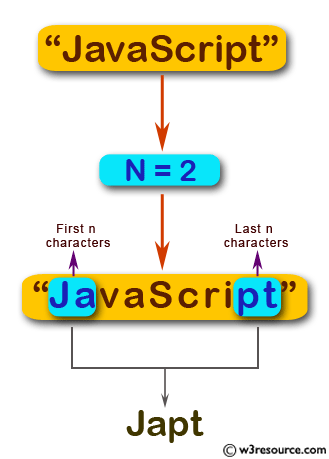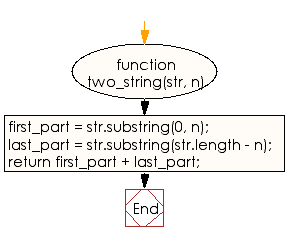# JavaScript: Create a new string taking the first and last n characters from a given string

## JavaScript Basic: Exercise-68 with Solution

Write a JavaScript program to create a new string taking the first and last n characters from a given string. The string length must be greater or equal to n.

Pictorial Presentation:Sample Solution:

HTML Code:

``````<!DOCTYPE html>
<html>
<meta charset="utf-8">
<meta name="viewport" content="width=device-width">
<title>JavaScript program to create a new string using the first and last n characters from a given string. The string length must be greater or equal to n.</title>
<body>

</body>
</html>
```
```

JavaScript Code:

``````function two_string(str, n)
{
first_part = str.substring(0, n);
last_part = str.substring(str.length - n);
return first_part + last_part;
}

console.log(two_string("JavaScript", 2));
console.log(two_string("JavaScript", 3));
```
```

Sample Output:

```Japt
Javipt
```

Flowchart:ES6 Version:

``````function two_string(str, n)
{
first_part = str.substring(0, n);
last_part = str.substring(str.length - n);
return first_part + last_part;
}

console.log(two_string("JavaScript", 2));
console.log(two_string("JavaScript", 3));
``````

Live Demo:

See the Pen Create a new string taking the first and last n characters from a given string by w3resource (@w3resource) on CodePen.

What is the difficulty level of this exercise?

Test your Programming skills with w3resource's quiz.

﻿

## JavaScript: Tips of the Day

Returns the sum of the powers of all the numbers from start to end (both inclusive)

Example:

```const sumPower = (end, power = 2, start = 1) =>
Array(end + 1 - start)
.fill(0)
.map((x, i) => (i + start) ** power)
.reduce((a, b) => a + b, 0);
console.log(sumPower(10)); // 385
console.log(sumPower(10, 3)); // 3025
console.log(sumPower(10, 3, 5)); // 2925
```

Output:

```385
3025
2925
```